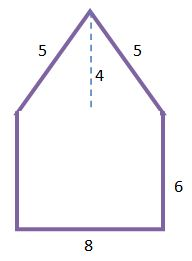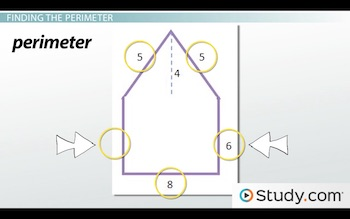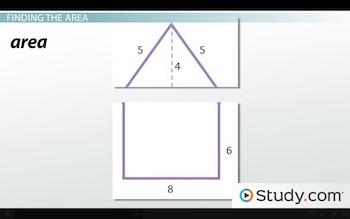# Combined Figures: Perimeter, Area, and Volume

An error occurred trying to load this video.

Try refreshing the page, or contact customer support.

Coming up next: Line Segments & Rays: Definition & Measurement

### You're on a roll. Keep up the good work!

Replay
Your next lesson will play in 10 seconds
• 0:01 Combined Figures
• 0:53 Finding the Perimeter
• 2:04 Finding the Area
• 3:42 Finding the Volume
• 4:57 Lesson Summary
Save Save

Want to watch this again later?

Timeline
Autoplay
Autoplay
Speed Speed Audio mode

#### Recommended Lessons and Courses for You

Lesson Transcript
Instructor: Yuanxin (Amy) Yang Alcocer

Amy has a master's degree in secondary education and has taught math at a public charter high school.

Watch this video lesson to learn how you can break up the shapes in a combined figure to easily find the perimeter, area, and volume of the whole figure.

## Combined Figures

We learn about triangles, squares, and circles in math. We know how to find the perimeter, the area, and the volume of each of these shapes on their own. But what happens when you have a combined figure, a shape that is the combination of several simpler shapes? Many shapes that we come across are combined figures. Look at your house, and you can see that you have a rectangular box plus a triangular box on top. How would you find the perimeter, the area, and the volume of such a figure? This is the question that we will be answering in this video lesson.

We will look at this combined figure that is the combination of a rectangle and a triangle:It kind of looks like a house. All the lengths are in feet, and we'll look at how to find the perimeter of this shape, the area of it, and also the volume of the shape when our shape is in three dimensions. The method that you will learn in this video lesson can be applied to any combined figure.

## Finding the Perimeter

We begin with finding the perimeter. Our shape looks like we drew a house on a piece of paper. To find the perimeter of our shape, we can highlight just the sides that make up the outside of our shape. Think of the outside as the fence that keeps our shape contained. So, we need to know the distance of this fence.

We need to know the length of each side. If we don't know the length of some of the sides, we need to figure them out. To find the length of some sides, we can look at the other sides to see if we can calculate them. For example, for our shape, we have four sides that are labeled with their lengths, but the fifth side isn't. However, because the fifth side is the other side of our triangle, we can calculate it, since this side is the same length as the side that is opposite it. In our case, 6 feet.Now we have all the lengths for our sides. So, all we have to do now is add up all these lengths to find our perimeter. Our perimeter is 5 + 5 + 6 + 8 + 6 = 30 feet. Notice how I've basically gone around the shape. This way I make sure that I am adding up all my sides. I recommend you do the same. Start with one side and then methodically work your way around the shape until you get back to the side you started with.

## Finding the Area

Next, we need to find the area of our combined figure. For this part, we want to separate our shape into its separate shapes. We already know that we have a rectangle with a triangle on top. So, what we can do is to draw a line to separate the two shapes. Now we can clearly see our two shapes:We do this to make finding our area easier. We can easily find the area of a rectangle as well as of a triangle. So, if we separate our combined figure into these easy shapes, then all we have to do is to find the area of each of our simple shapes and then add the areas up.

We first calculate the area of our rectangle. We know the area of a rectangle is its length multiplied by its width, so we have 6 times 8. This equals 48, so the area of our rectangle is 48 square feet. Next, we calculate the area of our triangle. The formula for the area of a triangle is half the base times the height: (1/2) * bh. Our base is the longer side of our triangle.

To unlock this lesson you must be a Study.com Member.

### Register to view this lesson

Are you a student or a teacher?

#### See for yourself why 30 million people use Study.com

##### Become a Study.com member and start learning now.
Back
What teachers are saying about Study.com

### Earning College Credit

Did you know… We have over 200 college courses that prepare you to earn credit by exam that is accepted by over 1,500 colleges and universities. You can test out of the first two years of college and save thousands off your degree. Anyone can earn credit-by-exam regardless of age or education level.